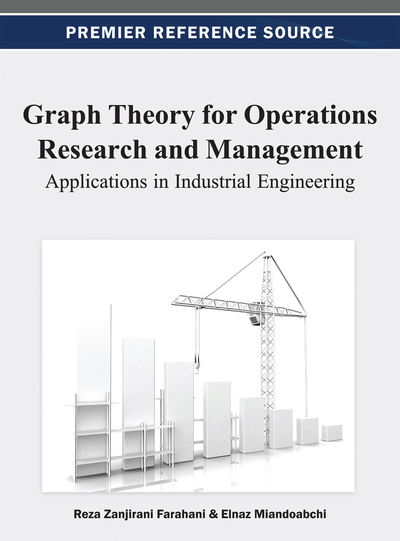# Planarity

Hossein Hojabri (Amirkabir University of Technology, Iran) and Elnaz Miandoabchi (Institute for Trade Studies and Research, Iran)
Copyright: © 2013 |Pages: 11
DOI: 10.4018/978-1-4666-2661-4.ch006
OnDemand PDF Download:
Available
\$37.50
No Current Special Offers

## Abstract

In this chapter the authors consider a property of a whole graph, which is of theoretical and practical importance. The concept addresses the possibility of drawing a given graph in a plane such that none of its edges intersect. The authors find this property, named planarity, very useful in application. They address the problem of planarity recognition including its theorems and its algorithm. Also, they discuss the concept of duality of graphs in the context of planarity.
Chapter Preview
Top

## Definitions Of Plane And Planar Graphs

### Definitions

• Embeddable graph: A graph G is called embeddable in a surface, when it is drawn on the surface such that no two edges of G cross each other.

• Planar graph: If a graph G can be embedded in the plane, then it is called planar.

• Plane graph: A graph G is a plane graph if it embedded in the plane.

• Nonplanar graph: If a graph G cannot be embedded in the plane, then it is called nonplanar.

Thus, any given plane graph is also planar. But a planar graph is not necessarily a plane graph as illustrated in Figure 1. In this Figure, both graphs are planar, but graph (a) is not a plane graph.

### Planarity Recognition

It is very important in many practical situations that we have an efficient procedure to recognize if a given graph is planar or not. In planarity testing, the question to answer is if an undirected graph can be embedded in a plane such that none of its edges intersect or not. In the past, some planarity testing algorithms in linear time have been presented by Hopcroft and Tajan (1974), and by Booth and Lueker (1976). However, their approaches are complicated. In order to simplify the planarity testing, some other algorithms have been devised. There is a reduction process we can use which simplifies any planarity test. In this approach, we eliminate any vertex of degree two from the graph by merging the two edges incident with the vertex. If this is done correctly, the resulting graph will have the same planarity status as the original graph under question. This reduction process is illustrated in Figure 2.

Once the mentioned reduction process has been taken into effect, the next obvious test is to check whether the number of edges of the graph under question is less than or equal tois the number of vertices of graph G. To continue the test, if the status of the graph is not still determined, we need the following concept.

Two graphs are called homeomorphic if one graph can be obtained from the other one with a creation of edges in series. Figure 3 illustrates two homeomorphic graphs.

The following two theorems are useful in planarity recognition.

• Theorem 1: A graph G is planar if and only if any of its homeomorphic graphs is planar.

The next useful theorem that recognizes planarity of a given graph is Kuratowski’s Theorem. But before introducing this theorem, we need to present some other basic theorems and definitions.

## Complete Chapter List

Search this Book:
Reset